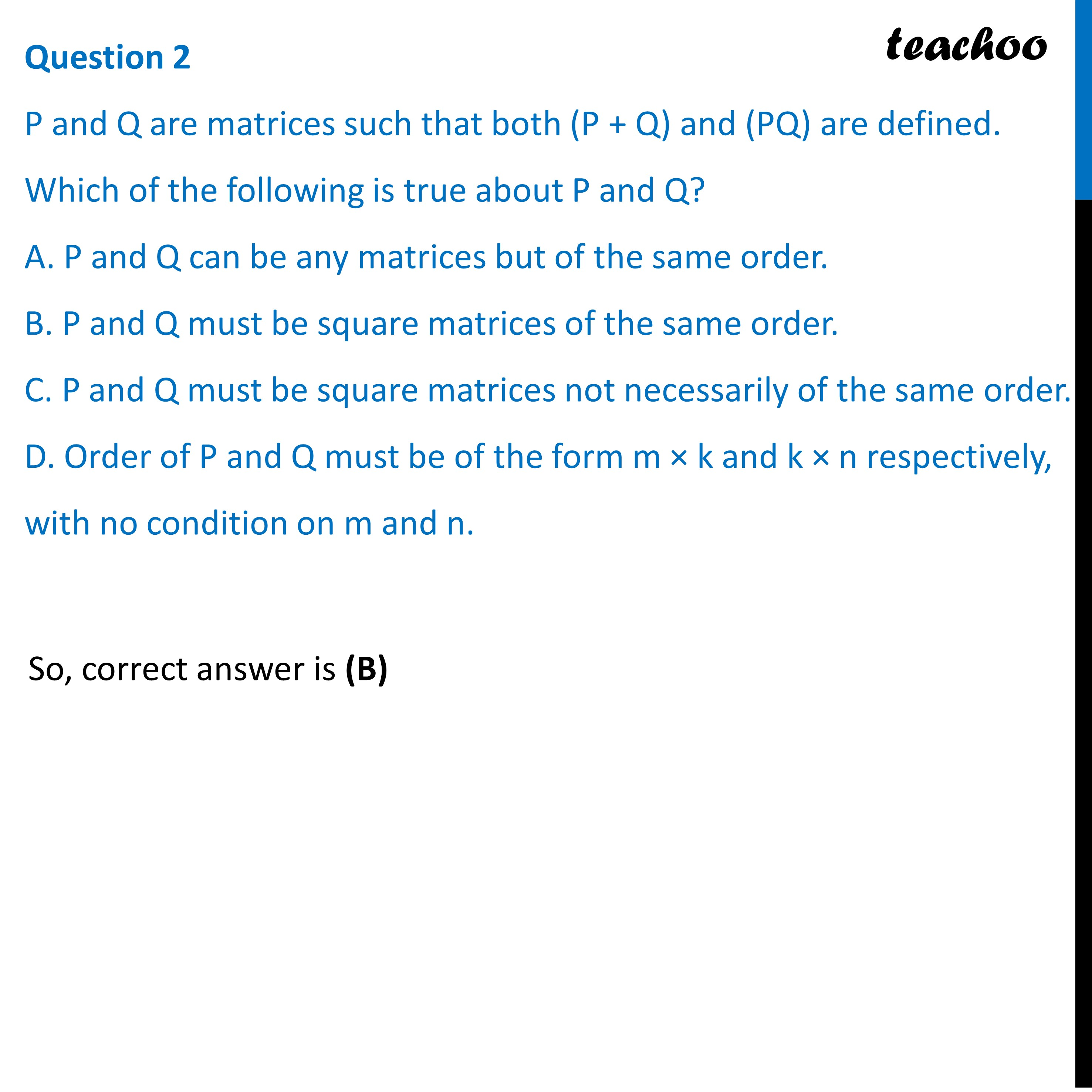Practice Questions CBSE - Maths Class 12 (2023 Boards)

Class 12
Solutions of Sample Papers and Past Year Papers - for Class 12 Boards

## D. Order of P and Q must be of the form m × k and k × n respectively, with no condition on m and n.Get live Maths 1-on-1 Classs - Class 6 to 12

### Transcript

Question 2 P and Q are matrices such that both (P + Q) and (PQ) are defined. Which of the following is true about P and Q? A. P and Q can be any matrices but of the same order. B. P and Q must be square matrices of the same order. C. P and Q must be square matrices not necessarily of the same order. D. Order of P and Q must be of the form m × k and k × n respectively, with no condition on m and n.So, correct answer is (B)